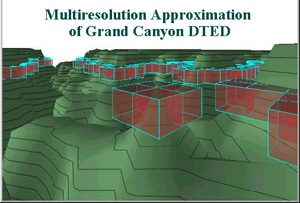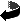MATH 758 S Wavelet and Multiresolution Analysis Spring 2003 Professor Robert Sharpley Meets: MWF  11:15-12:05 in LeConte College 316 Instructor Information  Office:   LeConte 313 D  Office Hours: MWF 10-11 a.m. and by appointment.Course Topics
The course will develop the basic principles and methods of (1) Fourier transforms on Rd, (2) wavelets, and (3) multiresolution analysis. Application of the concepts will be to partial differential equations, data compression, signal and image processing. Lectures will drawn from several general references (listed below) and will include the following topics:

An introduction to multiresolution analysis and a full development for the Haar and Stromberg wavelets in Lp(R) (1 < p < ∞); Fourier analysis on Rd, the L1 theory and computation of transforms for the Gaussian, Poisson, and heat kernels; the L2 theory and Plancherel theorem; the Lp theory and Hilbert transform (both as multiplier and singular integral); Heisenberg inequality, Poisson summation formula; band-limited functions and the Paley-Wiener Theorem; the sinc function and Shannon-Whittacker Sampling Theorem; development of the general Meyer-Mallat multiresolution analysis, scaling function and construction of wavelets; wavelets and Fourier transform criteria, Riesz's factorization lemma, Daubechies' construction of smooth compactly supported wavelets; Rademacher functions, probabilistic independence and unconditional convergence of series in Banach spaces, Khinchin's inequality, rearrangement and multipliers of series, wavelet bases as unconditional bases; DeVore's nonlinear approximation and Besov spaces; optimal entropy encoding in Lp; redundant approximation using Courant elements and surface compression.

Prerequisites
Fourier Analysis (Math 750)

Lectures:

General References

• I. Daubechies, "Ten Lectures on Wavelets," CBMS Regional Conference Series in Applied Mathematics # 61, SIAM, 1992. [QA403.3 .D38 1992]
• P. Wojtaszczyk, "A Mathematical Introduction to Wavelets", London Math. Soc. Student Text 37, Cambridge University Press, 1997.
• R.A. DeVore and V. Popov, Interpolation of Besov spaces, Trans. Amer. Math. Soc. 305(1988), 397-414.
• R.A. DeVore, B. Jawerth, and V. Popov, Compression of Wavelet Coefficients, Amer. J. Math. 114(1992), 737-785.
• R.A. DeVore, Nonlinear Analysis, Acta Numerica, 7(1998),51-150.
• A. Cohen, W. Dahmen, I. Daubechies, and R. A. DeVore, Tree Approximation and Encoding, ACHA 11(2001), 192-226.
• B. Karaivanov, P. Petrushev, and R.C. Sharpley, Algorithms for nonlinear piecewise polynomial approximation: Theoretical aspects, Trans. Amer. Math. Soc., to appear; IMI preprint 2002:13, University of South Carolina.
• B.S. Kashin and A.A. Saakyan, "Orthogonal Series," American Mathematical Society, Providence, RI, 1989. [QA404.5 .K3413 1989]
• C. Bennett and R. Sharpley, Interpolation of Operators," Academic Press, New York, 1988. (469 pp.) [QA3 .P8 vol. 129]
• M.A. Pinsky, "Introduction to Fourier Analysis and Wavelets", Brooks/Cole, Oct 2001. (432 pp.)This page maintained by Robert Sharpley (sharpley@math.sc.edu) and last updated Jan 2003.  This page ©2002-2003, The Board of Trustees of the University of South Carolina.  URL: http://www.math.sc.edu/~sharpley/math758S
Return to Sharpley's Home Page